# SVM -支持向量机原理与实践之实践篇

SVM -支持向量机原理与实践之实践篇

1. # 实验内容和目标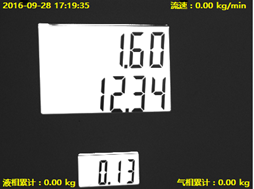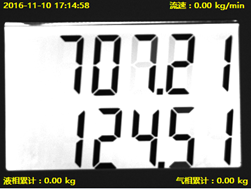1. # 准备工作

1. ## OpenCV 介绍

OpenCV是一个开源的计算机视觉库，它包含了一些完整的视觉处理算法的实现，当然还有一些机器学习的算法，例如SVM，决策树等。其中我们的讲解中就是用到它的SVM分类算法的实现。

1. ## 图像样本处理

1. ### 原始样本输入：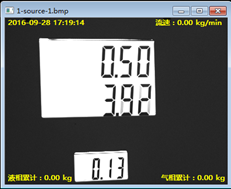1. ### 灰度化

cvtColor(src_in, grey, CV_BGR2GRAY);//转化为灰度图像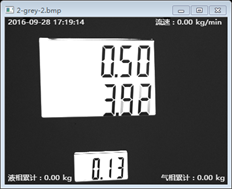1. ### 二值化

1. threshold(grey, grey, 0, 255, CV_THRESH_OTSU + CV_THRESH_BINARY_INV);
threshold(grey, grey, 0, 255, CV_THRESH_OTSU + CV_THRESH_BINARY_INV);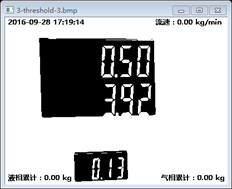1. ### 取轮廓

1. findContours(grey,
2. contours,// a vector of contours
3. CV_RETR_TREE,
4.                                     CV_CHAIN_APPROX_NONE); // all pixels of each contours
findContours(grey,
contours,// a vector of contours
CV_RETR_TREE,
CV_CHAIN_APPROX_NONE); // all pixels of each contours


取轮廓后我们再取ROI可以得到如下图所示的两个符合标准的图像的方框，由下面的细小白线框出：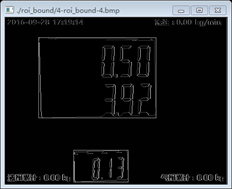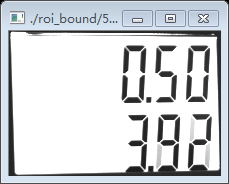1. ### 旋转校正

Rect mr = roi_rect.boundingRect();

1. Rect_<floatsafeBoundRect = Rect_<float>(mr.x, mr.y, mr.width, mr.height);
2. bound_mat = src_in(safeBoundRect);
Rect_<float> safeBoundRect = Rect_<float>(mr.x, mr.y, mr.width, mr.height);
bound_mat = src_in(safeBoundRect);


1. float roi_angle = roi_rect.angle;
2. Point2f roi_ref_center = roi_rect.center - safeBoundRect.tl();
3. rotation(bound_mat, rotated_mat, roi_rect.size, roi_ref_center, roi_angle);
float roi_angle = roi_rect.angle;
Point2f roi_ref_center = roi_rect.center - safeBoundRect.tl();
rotation(bound_mat, rotated_mat, roi_rect.size, roi_ref_center, roi_angle);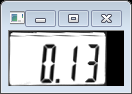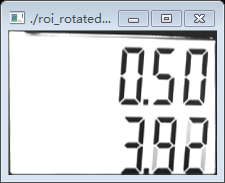1. ### 形态学开操作

morphologyEx(temp_mat, temp_mat, MORPH_OPEN, element);1. ### 阀值化1. ### 取轮廓

1. findContours(grey,
2.         contours,               // a vector of contours
3.         CV_RETR_EXTERNAL,       // retrieve the external contours
4.  CV_CHAIN_APPROX_NONE);  // all pixels of each contours
		            findContours(grey,
contours,               // a vector of contours
CV_RETR_EXTERNAL,       // retrieve the external contours
CV_CHAIN_APPROX_NONE);  // all pixels of each contours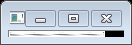1. ### 大小归一化

1. Mat cand_mat;
2. cand_mat.create(36, 136, CV_8UC3);
3. if (temp_cand_mat.cols >= 36 || temp_cand_mat.rows >= 136)
4.     resize(temp_cand_mat, cand_mat, cand_mat.size(), 0, 0, INTER_AREA);
5. else
6. resize(temp_cand_mat, cand_mat, cand_mat.size(), 0, 0, INTER_CUBIC);
			Mat cand_mat;
cand_mat.create(36, 136, CV_8UC3);
if (temp_cand_mat.cols >= 36 || temp_cand_mat.rows >= 136)
resize(temp_cand_mat, cand_mat, cand_mat.size(), 0, 0, INTER_AREA);
else
resize(temp_cand_mat, cand_mat, cand_mat.size(), 0, 0, INTER_CUBIC);1. # SVM模型训练

1. ## 贴标签

开始用SVM做训练之前，我们必须把处理后的样本分为两类，一类是有效的图片，另一类是无效的图片，然后从这两类图片中分别取出一部分用来做SVM模型训练，然后另一部分用来做测试集，这个测试集中同样包含有效的图片和无效的图片，用以验证SVM模型训练号的分类效果。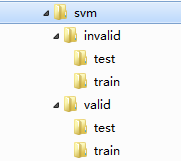1. ## 样本特征提取

 特征 描述 HOG 即Histogram of Oriented Gradient, HOG特征是一种在计算机视觉和图像处理中用来进行物体检测的特征描述子。它通过计算和统计图像局部区域的梯度方向直方图来构成特征。 LBP 即Local Binary Pattern，局部二值模式，是一种用来描述图像局部纹理特征的算子；它具有旋转不变性和灰度不变性等显著的优点。 HAAR Haar特征分为三类：边缘特征、线性特征、中心特征和对角线特征，组合成特征模板。

1. ## SVM参数说明

SVM类型：SVM设置类型(默认0) 　　0 -- C-SVC 　　1 --v-SVC 　　2 – 一类SVM 　　3 -- e -SVR 　　4 -- v-SVR 　　-t 核函数类型：核函数设置类型(默认2) 　　0 – 线性：u'v 　　1 – 多项式：(r*u'v + coef0)^degree 　　2 – RBF函数：exp(-gamma|u-v|^2) 　　3 –sigmoid：tanh(r*u'v + coef0)

-d degree：核函数中的degree设置(针对多项式核函数)(默认3) 　　-g r(gama)：核函数中的gamma函数设置(针对多项式/rbf/sigmoid核函数)(默认1/ k) 　　-r coef0：核函数中的coef0设置(针对多项式/sigmoid核函数)((默认0) 　　-c cost：设置C-SVC，e -SVR和v-SVR的参数(损失函数)(默认1) 　　-n nu：设置v-SVC，一类SVM和v- SVR的参数(默认0.5) 　　-p p：设置e -SVR 中损失函数p的值(默认0.1) 　　-m cachesize：设置cache内存大小，以MB为单位(默认40) 　　-e eps：设置允许的终止判据(默认0.001) 　　-h shrinking：是否使用启发式，0或1(默认1) 　　-wi weight：设置第几类的参数C为weight*C(C-SVC中的C)(默认1) 　　-v n: n-fold交互检验模式，n为fold的个数，必须大于等于2 　　其中-g选项中的k是指输入数据中的属性数。option -v 随机地将数据剖分为n部

1. ## 开始训练

1. void svm_train_test(void)
2. {
3. //#define AutoTrain
4.     svm_ = cv::ml::SVM::create();
5.     svm_->setType(cv::ml::SVM::C_SVC);
6.     svm_->setKernel(cv::ml::SVM::RBF);
7.     auto train_data = tdata();
8.
9. #ifndef AutoTrain
10.     double v_gamma = svm_->getGamma();
11.     double v_C = svm_->getC();
12.     fprintf(stdout,">> Training SVM RBF model gamma = %f C = %f, please wait...\n", v_gamma, v_C);
13. #else
14.     svm_->trainAuto(train_data, 10, svm_->getDefaultGrid(svm_->C),
15.     svm_->getDefaultGrid(svm_->GAMMA), svm_->getDefaultGrid(svm_->P),
16.     svm_->getDefaultGrid(svm_->NU), svm_->getDefaultGrid(svm_->COEF),
17.     svm_->getDefaultGrid(svm_->DEGREE),true);
18.     double v_gamma = svm_->getGamma();
19.     double v_coef0 = svm_->getCoef0();
20.     double v_C = svm_->getC();
21.     double v_Nu = svm_->getNu();
22.     double v_P = svm_->getP();
23.
24.     fprintf(stdout,">> Auto Training SVM paramter gamma %f,coef0 %f C %f Nu %f P %f\n", v_gamma, v_coef0, v_C, v_Nu, v_P);
25.     system("pause");
26.
27. #endif
28.     do    {
29.         svm_->setGamma(v_gamma);
30.         svm_->setC(v_C);
31.         long start =utils::getTimestamp();
32.         svm_->train(train_data);
33.         long end =utils::getTimestamp();
34.         fprintf(stdout,">> Training done. Time elapse: %ldms\n", end - start);
void svm_train_test(void)
{
//#define AutoTrain
svm_ = cv::ml::SVM::create();
svm_->setType(cv::ml::SVM::C_SVC);
svm_->setKernel(cv::ml::SVM::RBF);
auto train_data = tdata();

#ifndef AutoTrain
double v_gamma = svm_->getGamma();
double v_C = svm_->getC();
fprintf(stdout,">> Training SVM RBF model gamma = %f C = %f, please wait...\n", v_gamma, v_C);
#else
svm_->trainAuto(train_data, 10, svm_->getDefaultGrid(svm_->C),
svm_->getDefaultGrid(svm_->GAMMA), svm_->getDefaultGrid(svm_->P),
svm_->getDefaultGrid(svm_->NU), svm_->getDefaultGrid(svm_->COEF),
svm_->getDefaultGrid(svm_->DEGREE),true);
double v_gamma = svm_->getGamma();
double v_coef0 = svm_->getCoef0();
double v_C = svm_->getC();
double v_Nu = svm_->getNu();
double v_P = svm_->getP();

fprintf(stdout,">> Auto Training SVM paramter gamma %f,coef0 %f C %f Nu %f P %f\n", v_gamma, v_coef0, v_C, v_Nu, v_P);
system("pause");

#endif
do    {
svm_->setGamma(v_gamma);
svm_->setC(v_C);
long start =utils::getTimestamp();
svm_->train(train_data);
long end =utils::getTimestamp();
fprintf(stdout,">> Training done. Time elapse: %ldms\n", end - start);   
1.         fprintf(stdout,">> Saving model file...\n");
2.         svm_->save(svm_xml_);
3.         fprintf(stdout,">> Your SVM Model was saved to %s\n", svm_xml_);
4.         fprintf(stdout,">> Testing...\n");
5.         svm_test_tmp(v_gamma, v_C, start, end);
6. #ifndef AutoTrain
7.         v_gamma += 0.2;
8.         v_C += 0.2;
9. #else
10.         break;
11. #endif
12.     }while (v_gamma && v_C 30.0);
13. }
        fprintf(stdout,">> Saving model file...\n");
svm_->save(svm_xml_);
fprintf(stdout,">> Your SVM Model was saved to %s\n", svm_xml_);
fprintf(stdout,">> Testing...\n");
svm_test_tmp(v_gamma, v_C, start, end);
#ifndef AutoTrain
v_gamma += 0.2;
v_C += 0.2;
#else
break;
#endif
}while (v_gamma && v_C < 30.0);
}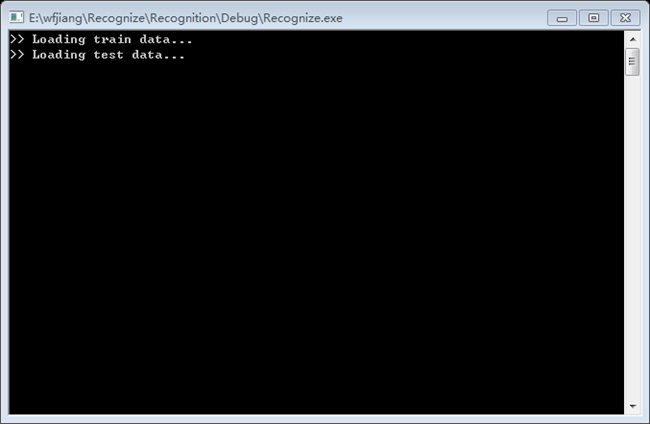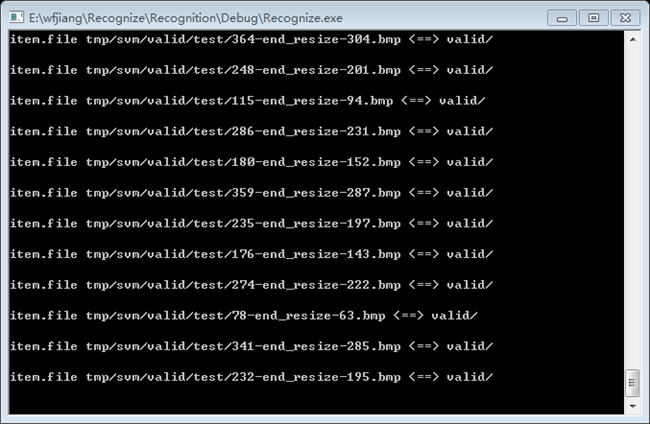1. ## SVM参数调优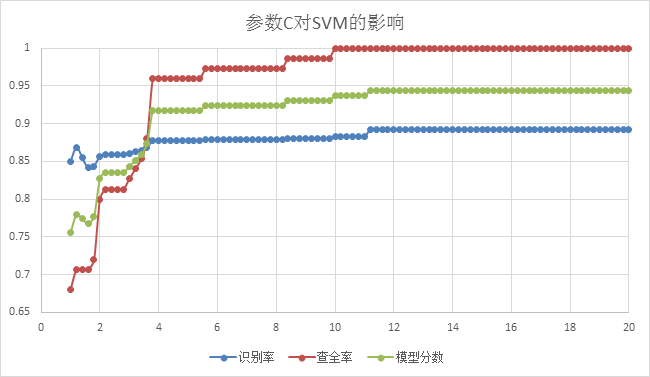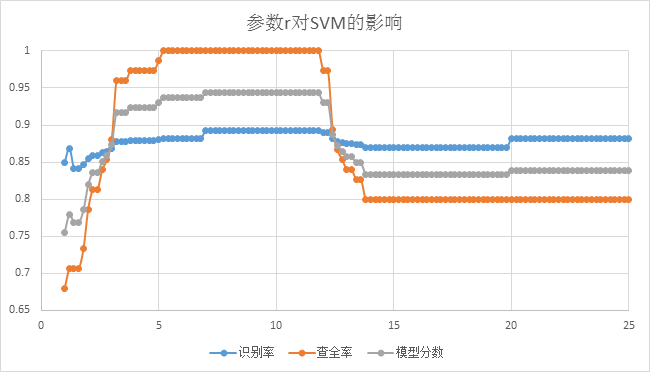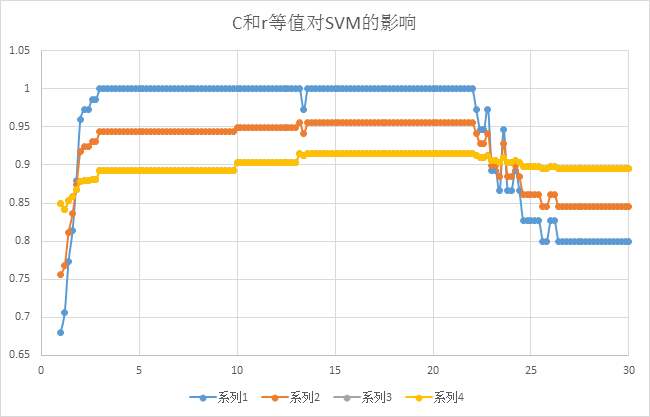posted @ 2017-03-31 00:15  wfjiang  阅读(8519)  评论(0编辑  收藏  举报